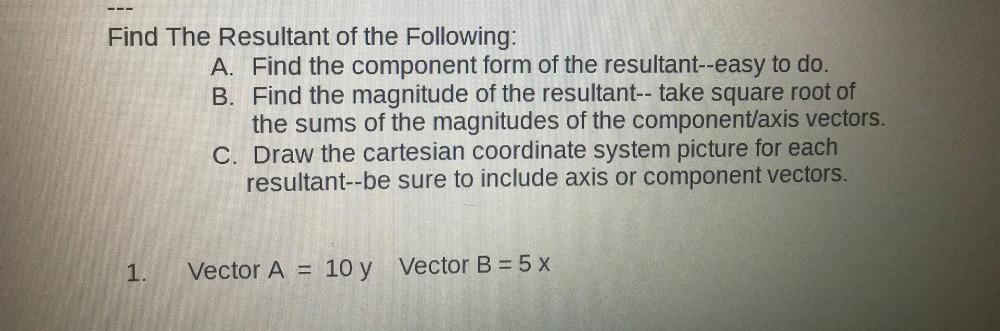Question:

# Find The Resultant of the Following: A. Find the component form of the resultant--easy to do. B. Find the magnitude of the resulFind The Resultant of the Following: A. Find the component form of the resultant--easy to do. B. Find the magnitude of the resultant-- take square root of the sums of the magnitudes of the component/axis vectors. C. Draw the cartesian coordinate system picture for each resultant--be sure to include axis or component vectors. 1. Vector A= 10 y Vector B = 5 x